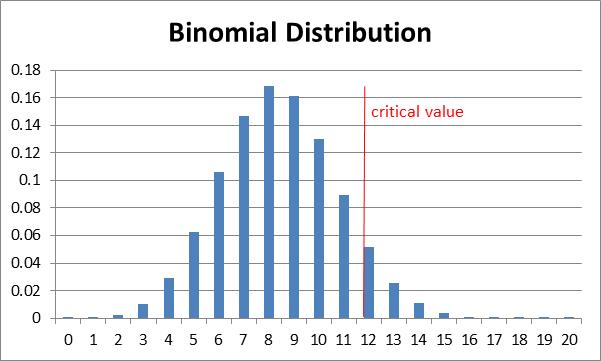# Statistical Power for the Binomial Distribution

### Power of one-tailed test

Example 1: What is the power of the test in Example 3 of Hypothesis Testing for the Binomial Distribution?

For this example we found 13 successes in a sample of size 24 and used a one-tailed test with α = .05 based on the binomial distribution with null and alternative hypotheses:

H0p ≤ .35
H1p > .35

As in Statistical Power of a Sample, to find the power of this test we must first calculate the critical value. This is done using the formula

xcrit = CRITBINOM(24, .35, 1−.05) = 12

This means that at least 95% of the distribution occurs for values x ≤ 12.Figure 1 – Histogram of the distribution

In fact, 95.8% of the distribution is to the left of the critical value (inclusive) since

BINOMDIST(xcrit, n, p, TRUE) = BINOMDIST(12, 24, .35, TRUE) = .9577

The power of the test is calculated using the following formula where pobs = 13/24 = .54167:

1 – BINOMDIST(xcrit, n, pobs, TRUE) = 1 – BINOMDIST(12, 24, .54167, TRUE) = 58.30%

We can chart the power of the test for various values of pobs as shown in Figure 2.Figure 2 – Power Curve, one-tailed test

Here cell N11 contains the formula =BINOMDIST(\$O\$8,\$O\$6,M11,TRUE) and O11 contains the formula =1−N11. The rest of the table is created by highlighting the range N11:O23 and pressing Ctrl-D.

### Power of two-tailed test

Example 2: Repeat Example 1 for a two-tailed test.

This time there are two critical values: one on the right (x+crit) and one on the left (x-crit).

These are calculated as follows:

x+crit = CRITBINOM(24, .35, 1−.05/2) = 13
x-crit = CRITBINOM(24, .35, .05/2) = 4

The power of the test is calculated using the following formula where pobs= 13/24 = .54167:

= 1 + BINOMDIST(x-crit−1, npobs, TRUE) – BINOMDIST(x+crit, n, pobs, TRUE)
= 1 + BINOMDIST(3, 24, .54167, TRUE) – BINOMDIST(13, 24, .54167, TRUE)            = 42.15%

Real Statistics Function: The Real Statistics Resource Pack provides the following function to calculate statistical powert automatically.

BINOM_POWER(p0, p1, n, tails, α) = the power of a one sample binomial test when p0 = probability of success on a single trial based on the null hypothesis, p1 = expected probability of success on a single trial, n = the sample size, tails = # of tails: 1 or 2 (default) and α = alpha (default .05).

Referring to Figure 2, we see that BINOM_POWER(.35, .45, 24, 1, .05) = .242. Referring to Figure 3, we see that BINOM_POWER(.35, .45, 24, 2, .05) = .134

Statistics for Beginners in Excel – Confidence Intervals for Sampling Distributions

# Personal Career & Learning Guide for Data Analyst, Data Engineer and Data Scientist

## Applied Machine Learning & Data Science Projects and Coding Recipes for Beginners

A list of FREE programming examples together with eTutorials & eBooks @ SETScholars

# Projects and Coding Recipes, eTutorials and eBooks: The best All-in-One resources for Data Analyst, Data Scientist, Machine Learning Engineer and Software Developer

Topics included: Classification, Clustering, Regression, Forecasting, Algorithms, Data Structures, Data Analytics & Data Science, Deep Learning, Machine Learning, Programming Languages and Software Tools & Packages.
(Discount is valid for limited time only)`Disclaimer: The information and code presented within this recipe/tutorial is only for educational and coaching purposes for beginners and developers. Anyone can practice and apply the recipe/tutorial presented here, but the reader is taking full responsibility for his/her actions. The author (content curator) of this recipe (code / program) has made every effort to ensure the accuracy of the information was correct at time of publication. The author (content curator) does not assume and hereby disclaims any liability to any party for any loss, damage, or disruption caused by errors or omissions, whether such errors or omissions result from accident, negligence, or any other cause. The information presented here could also be found in public knowledge domains.`

# Learn by Coding: v-Tutorials on Applied Machine Learning and Data Science for Beginners

Please do not waste your valuable time by watching videos, rather use end-to-end (Python and R) recipes from Professional Data Scientists to practice coding, and land the most demandable jobs in the fields of Predictive analytics & AI (Machine Learning and Data Science).

The objective is to guide the developers & analysts to “Learn how to Code” for Applied AI using end-to-end coding solutions, and unlock the world of opportunities!